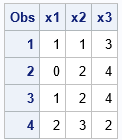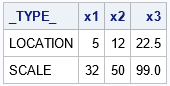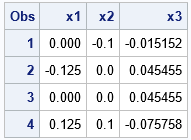You can standardize a numerical variable by subtracting a location parameter from each observation and then dividing by a scale parameter. Often, the parameters depend on the data that you are standardizing. For example, the most common way to standardize a variable is to subtract the sample mean and divide by the sample standard deviation. For a variable X, let c = mean(X) and s = std(X). Then one way to standardize X is to form the new variable
Y = (X - c) / s
which is a vector shorthand for the variable Y whose i_th observation is yi = (xi - c) / s.

Ironically, there are many ways to standardize a variable. You can use many different statistics to obtain estimates for c and s. You might want to do this is because the mean and standard deviation are not robust to the presence of outliers. For some data, a robust estimate of location and scale might be more useful. For example, you could choose the sample median as the location parameter (c) and a robust estimate of scale for the scale parameter (s). Robust estimates of scale include the interquartile range, the MAD statistic, and a trimmed standard deviation, to name a few.

When I want to standardize a set of variables, I use PROC STDIZE in SAS. It is a powerful tool that includes many built-in methods for standardizing variables. I previously wrote an article that shows several ways to use PROC STDIZE to standardize data.

You might not know that PROC STDIZE supports a way to perform your own custom standardizations. That is, PROC STDIZE supports nonstandard standardizations! Briefly, you can use any method to estimate the location and scale parameters for each variable and write those estimates to a SAS data set. You can then use the METHOD=IN(DataName) option on the PROC STDIZE statement to use those special estimates to standardize the variables. This article describes how to use the METHOD=IN option to perform a nonstandard standardization.

### The structure of the IN= data set

The IN= data set for PROC STDIZE must be in a specific format. Suppose you want to standardize three numerical variables: X1, X2, and X3. The IN= data set must contain variables that have the same names. The data set must also contain a character variable, _TYPE_. The rows specify parameters for each variable, and the _TYPE_ variable indicates which parameter is specified. For example, the following DATA step defines example data. A second data set specifies the location and scale parameters for each variable by using the format that is required by PROC STDIZE. In this example, the location and scale parameters for the X1 variable are 1 and 4, respectively. The parameters for the X2 variable are 2 and 5. The parameters for X3 are 3 and 6.

```/* specify the location and scale parameters for each var */ data Parms; length _TYPE_ \$14; input _TYPE_ /* required variables */ x1 x2 x3; /* names of variables that you are going to standardize */ datalines; LOCATION 1 2 3 SCALE 4 5 6 ;```

If you create a data set that contains variables X1, X2, and X3, you can use the parameters in the Parms data set to center and scale the variables, as follows:

```/* the raw data to be standardized */ data Have; input x1 x2 x3; datalines; 5 7 21 1 12 27 5 12 27 9 17 15 ;   /* use parameters in data set to standardize X1-X3 */ proc stdize data=Have out=Want method=in(Parms); var x1 x2 x3; run; proc print data=Want; run;```The call to PROC STDIZE standardizes the variables by using the specified parameters. For example, the X2 variable is transformed according to the formula (X2 - 2)/5.

### Create an IN= data set from PROC MEANS

The previous example hard-coded the location and scale parameters for each variable. In practice, you often estimate the parameters by using statistics from procedures such as PROC SUMMARY, PROC MEANS, or PROC IML. This section shows how to create the METHOD=IN data set from the PROC MEANS output.

Suppose that you want the location parameter to be the sample means and the scale parameter to be the corrected sum of squares Σi (xi - m)2, where m is the sample mean. This combination of parameters is not one of the methods that are built into PROC STDIZE. However, you can use the METHOD=IN option to implement it.

First, use PROC MEANS to compute the means and corrected sum of squares (CSS). The statistics are in one long row. You can use a DATA step and arrays to reshape the data into the form required by PROC STDIZE:

```%let varNames = x1 x2 x3; %let locNames = c1-c3; %let scaleNames = s1-s3; proc means data=Have noprint; var &varNames; output out=Stats(drop=_TYPE_) mean=&locNames /* using mean to estimate location */ css=&scaleNames; /* using CSS for scale */ run;   /* the statistics are in one long row. Make one row for each statistic */ data Parms; length _TYPE_ \$14; array x[*] &varNames; /* new column names */ array loc[*] &locNames; /* column names for location parameter */ array scale[*] &scaleNames; /* column names for scale parameter */ set Stats; _TYPE_ = "LOCATION"; do i=1 to dim(loc); x[i] = loc[i]; end; output; _TYPE_ = "SCALE"; do i=1 to dim(scale); x[i] = scale[i]; end; output; keep _TYPE_ &varNames; run;   proc print data=Parms noobs; run;```For each variable, the location parameter is the sample mean. The scale parameter is the sample CSS. As before, you can use these values to standardize the raw data:

```/* use parameters in data set to standardize X1-X3 */ proc stdize data=Have out=Want2 method=in(Parms); var &varNames; run; proc print data=Want2; run;```### Summary

This article shows how to use PROC STDIZE in SAS to perform "nonstandard" standardizations. PROC STDIZE supports the METHOD=IN option, which enables you to specify a data set that contains the location and scale parameters. You can use SAS procedures (such as PROC MEANS) to compute and statistics you want. By writing the statistics to a data set and adding a _TYPE_ variable, you can standardize the variables by using any estimate of location and scale.

The post Nonstandard ways to standardize variables appeared first on The DO Loop.